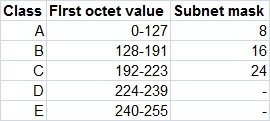TCP/IP defines five classes of IP addresses: class A, B, C, D, and E. Each class has a range of valid IP addresses. The value of the first octet determines the class. IP addresses from the first three classes (A, B and C) can be used for host addresses. The other two classes are used for other purposes – class D for multicast and class E for experimental purposes.

The system of IP address classes was developed for the purpose of Internet IP addresses assignment. The classes created were based on the network size. For example, for the small number of networks with a very large number of hosts, the Class A was created. The Class C was created for numerous networks with small number of hosts.For the IP addresses from Class A, the first 8 bits (the first decimal number) represent the network part, while the remaining 24 bits represent the host part. For Class B, the first 16 bits (the first two numbers) represent the network part, while the remaining 16 bits represent the host part. For Class C, the first 24 bits represent the network part, while the remaining 8 bits represent the host part.

• 10.50.120.7 – because this is a Class A address, the first number (10) represents the network part, while the remainder of the address represents the host part (50.120.7). This means that, in order for devices to be on the same network, the first number of their IP addresses has to be the same for both devices. In this case, a device with the IP address of 10.47.8.4 is on the same network as the device with the IP address listed above. The device with the IP address 11.5.4.3 is not on the same network, because the first number of its IP address is different.
• 172.16.55.13 – because this is a Class B address, the first two numbers (172.16) represent the network part, while the remainder of the address represents the host part (55.13). A device with the IP address of 172.16.254.3 is on the same network, while a device with the IP address of 172.55.54.74 isn’t.

NOTE
The system of network address ranges described here is generally bypassed today by use of the Classless Inter-Domain Routing (CIDR) addressing.

Special IP address ranges that are used for special purposes are:

• 0.0.0.0/8 – addresses used to communicate with the local network